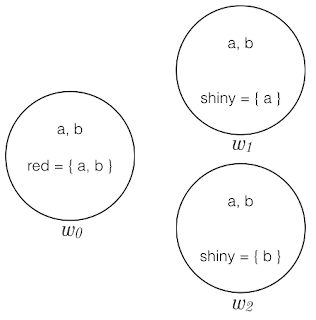(1). It is possible for every red thing to be shiny

(1a). $\Diamond\forall x (Rx\to Sx)$ （有可能所有東西都是：如果是紅色的，便有光澤）
(1b). $\forall x \Diamond(Rx\to Sx)$ （每個東西都有可能是：如果是紅色則有光澤）
(1c). $\forall x(Rx\to\Diamond Sx)$ （每個紅色的東西都可能有光澤）Fig. 1Fig. 2

(1c) 說每個紅色的東西都有可能有光澤，基準世界是 $w_0$ ，故 (1c) 說的每個紅色的東西是指 $w_0$ 裡的 a 和 b 。由於 a 在 $w_1$ 有光澤， b 在 $w_2$ 有光澤，所以確是每個紅色的東西都可能有光澤。然而， (1) 的意思是： $w_0$ 的紅色東西有可能全部一齊都有光澤。這情況底下，沒有一個可能世界是 a, b 一齊有光澤，因此 (1c) 同樣無法捕捉 (1) 的意思。

(1d). $\Diamond\forall x(\mathcal{A}Rx\to Sx)$

$v(p)=\sf{T}$

$v(p, w)=\sf{T}$

$v(p, w_0, w)=\sf{T}$

Kripke (1959) A Completeness Theorem in Modal Logic. JSL
Kripke (1963) Semantical Analysis of Modal Logic I Normal Modal Propositional Calculi. MLQ
Segerberg (1973) Two-Dimensional Modal Logic. JPL
Crossley & Humberstone (1977) The Logic of ‘Actually’. RML
Davies & Humberstone (1980) Two Notions Of Necessity. PS

4 則留言:

1.「有可能，紅色的東西全部都是有光澤的東西」和「有可能所有紅色的東西都有光澤」這兩句中文太相似，可以被理解為同一意思（我看不到「全部」和「所有」在意思上有甚麼不同），不宜用來分別表達（1）和（1a）。我會這樣寫：

（1）有可能所有紅色的東西一起都有光澤。
（1a）有可能所有東西都是：如果是紅色的，便有光澤。

1.已改，多謝！

2.1.